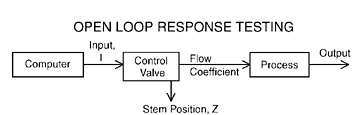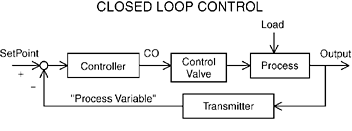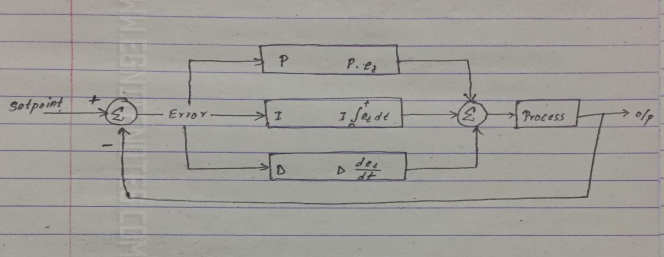### Notes of Embedded System [CT 655]

#### Control System

Open Loop and Closed Loop Control System

Control System

Control system is a system designed to control physical system output to a desired limit by setting desired reference output.

-Automobile Cruise Controller
This controller seeks to make a car’s speed track a desired speed by setting the car’s throttle and brake inputs by setting the car’s throttle and brake inputs.

-Thermostat controller
It forces a building temperature to a desired temperature by turning on the heater or Air Conditioning System and adjusting the fan speed.

Open Loop Control System

- It is a feed forward control system

-It consists of following parts:

1. Plant process
-It is the physical system to be controlled.

2. Output
-It is the particular aspect of physical system that we intend to control.

3. Reference input
-It is the desired value that we want to see for the output.

4. Actuator
-It is the device used to control i/p to the plant.

5. Controller
-It is the system used to compute the i/p to the plant.

6. Disturbance
-It is an additional undesirable i/p imposed by environment that causes o/p to differ from out expectation.Designing Open Loop control system

Model of plant
Model of the plant is developed to make design phase easier and to desire the controller. For ex Throttler
That goes from 0 to 45 degrees.
-On flat surface at 50 mph ,open throttle to 40 degree.
-Wait 1 “time unit"
-Measure the speed , lets say 55 mph
-Then the equation that holds for all scenarios gives model of the plant.

```v(t+1) = 0.7 v(t) + 0.5 u(t)
55 = 0.7 * 50 + 0.5 * 40
```

Controller design
Controller provides a specific rule to achieve the goal of the control system.

```Assume we have a linear function:
U(t) = F[r(t)] = P*r(t)
where, P = constant ,
r(t)  = desired speed..
Now,
v(t+1)  = 0.7*v(t)  + 0.5*u(t)  =  0.7*v(t) + 0.5*P*r(t) ```

Let v(t+1) = v(t); at steady state = v(ss) ;
v(ss) = 0.7*v(ss) + 0.5*P*r(t)

At steady state we want v(ss) = r(t)

1= 0.7+0.5*P
P = 0.6

Hence the control rule is defined as:
u(t) = P*r(t) = 0.6* r(t)

Analyzing Controller

```Suppose,
v(0)  = 20mph and r(0)  = 50mph ```

Then , vt+1 = 0.7 *v(t) + 0.5*0.6*r(t)
=0.7*v(t) + 0.3*50
=0.7*v(t) + 15

Therefore throttle position = 0.6*50 = 30 degree

Considering Disturbance

`Assuming road grade affect speed from -5mph to +5 mph`

Time v(t) v(t) [w = +5] v(t) [w = -5]
0 20 20 20
1 29 24 34
2 35.3 26.80 43.80
3 39.71 28.76 50.66
4 42.80 30.13 55.46
5 44.96 31.09 58.82
6 46.47 31.76 61.18
7 47.53 32.24 62.82
8 48.27 32.56 63.98
9 48.79 32.80 64.78
10 49.15 32.96 65.35
11 49.41 33.07 65.74
12 49.58 33.15 66.02

Determining performance

```     v(t+1) =  0.7*v(t)  + 0.5*u(t)   - w(0)
v(1) = 0.7*v(0)  + 0.5*u(0)   - w(0)
v(2)  = 0.7(0.7*v(0)  + 0.5*u(0)   - w(0)) + 0.5P * r(0) – w(0)
v(t)  =0.72v(0) + ( 0.7^(t-1) + 0.7^(t-2)+ ……….. +0.7 + 1)(0.5P*r(0) – w(0))
```

- Coefficient of v(t) determines rate of decay of v(0)
- If > 1 or < -1 , v(t) will grow without bound
- Otherwise v(t) will oscillate.

Closed Loop Control System

-It is a feedback control system.
-It minimizes tracking error.
-It consists of some additional part (sensors to measure plant o/p and error detector to detect errors and mistakes in the data).Designing Closed Loop Control System

Design model of plant

```     v(t+1) =  0.7*v(t)  + 0.5*u(t)   - w(t)
```

Design Controller

```
U(t)  =  P(r(t)  - v(t) )```

v(t+1) = 0.7*v(t) + 0.5 P(r(t) - v(t)) - w(t)
= (0.7- 0.5P)v(t) + 0.5P * r(t) - w(t)

v(t) = (0.7- 0.5P)^t * v(0) + { (0.7- 0.5P)^(t-1) + (0.7- 0.5P)^(t-2) + …… + (0.7- 0.5P) +1 } ( 0.5 P * r(0) - w(0))

So,
Stability constraint requires:
|0.7 – 0.5P| <1
Or, -1< 0.7 -0.5P <1
Thus, -0.6

Reducing effect of v0
To reduce the effect of initial condition (i.e. v0) ,
a) 0.7 – 0.5p must be as small as possible.
i.e. 0.7-0.5p = 0 ;
thus, p = 1.4

Avoid Oscillation
To avoid oscillation, 0.7-0.5p must be greater than or equal to 0.
i.e. 0.7 – 0.5p >= 0
therefore, p<=1.4

Perfect Tracking

`Assume at steady state v(ss)  , v(t+1)  = v(t)  ;`

V(ss) = (0.7 -0.5p)v(ss) + 0.5 P * r(0) – w(0)
Or (1-0.7+0.5p)v(ss) = 0.5 P * r(0) - w(0)
So, v(ss) = (0.5 P * r(0) - w(0)) / (0.3+ 0.5p)
= 0.5 / (0.5 P * r(0) - w(0)) * r(0) - 1 / (0.3+ 0.5p) * w0

To make vss close to r0 ­ , p should be as large as possible.

Analyse controller
-set P=3.3 , saturate at 0.45, oscillation
-set p=1 to avoid oscillation . Terrible SS performance.

Control System and PID Controller

P controller

- It multiplies the tracking error by a constant.
- u(t) = P[r(t) – v(t)]
- P affects transient response (stability ),steady state tracking error and disturbance rejection .

PD controller

- It considers the size of error over time.
- It keeps track of error derivative.
- u(t) = P*e(t) + delta*[e(t) – e(t-1))
- P set for best tracking and disturbance control.
- D set to control oscillation and convergence rate

PI controller

- It sum up error over time.
- u(t) =P*e(t) + I* (e(0) + e(1) + …… + e(t))
- P controls disturbance.
- I ensures steady state convergence.

PID Controller

- Combines proportional , integral and derivative control.
- u(t) = P*e(t) + delta*[e(t) – e(t-1)) + I* (e(0) + e(1) + …… + e(t))
- It achieves desired stable transient behavior.

The block diagram of PID Controller is shown below:Software Coding of a PID Controller

Code in C:

`//Sample Coding for PID controller`

..including different library files..

void main() {
double sen_val,act_val,err_current;
PID_DATA pid_data;
PidInitialize(&pid_data);
while(1)
{
sen_val = SensorGetValue();
ref_val= ReferenceGetValue();
act_val = PidUpdate(&pid_data,sen_value,ref_val);
ActuatorSetValue(act_value);
}

}

typedef struct PID_DATA {
double Pgain,Dgain,Igain ;
double sen_val_previous ; //Find derivative
double error_sum ; //cumulative error
}

//definition of function PidUpdate
double PidUpdate(PID_DATA * pid_data , double sen_val,double ref_val)
{
double Pterm,Iterm,Dterm;
double err,diff;
err = ref_val – sen_val ;
Pterm =pid_data->Pgain* err;
pid_data ->error_sum+= error;
Iterm =pid_data ->Igain*pid_data->error_sum ;
diff=pid_data->sen_val_previous = sen_val;
Oterm = pid_data ->Dgain* diff;
return (Pterm + Iterm+Dterm);
}

PID Tuning

-Pid tuning is the process of obtaining P,I and delta by ad-hoc methods.
-It is necessary because:
a) with analytics , the plant model may be complex .
b) we may not even have a plant model

Algorithm for PID Tuning:

2. Increase D until seeing oscillation
-Decrease delta a bit

3. Increase P until seeing oscillation
-Decrease P a bit

4. Increase I, until seeing oscillation

5. Repeat from second step until performance can be improved.

Issues with computer based control

1)Quantization and overflow

-quantization occurs when a signal must be altered to fit memory constraints.
-arithmetic operations cause quantization.
-e,g, assume an operation results 0.36 but it must be stored as 4 bit fractional number , so 0.25 is choosed instead of 0.36
-Overflow occurs when operation outputs a number with magnitude too large to be represented by a processor and thus chances of error.
-Uses fixed point representation carefully(time consuming)
-Uses floating point and co-processor(expensive)

2)Aliasing

-Aliasing is due to the discrete nature of sampling.
- Example : Sampling at 2.5 Hz, period of 0.4;
y(t) = 1.0 * sin (6 * pi * t), f = 3Hz
y(t) = 1.0 * sin (pi * t), f = 0.5Hz
These two functions are indistinguishable.

3) Computational Delay

- The computation causes delay to occur that causes actuation to delay.
- The implementation delay must be negligible.
- Software delay is hard to predict.

Benefits of Computer Based Control

1. Cost
2. Programmable
3. Reproducible